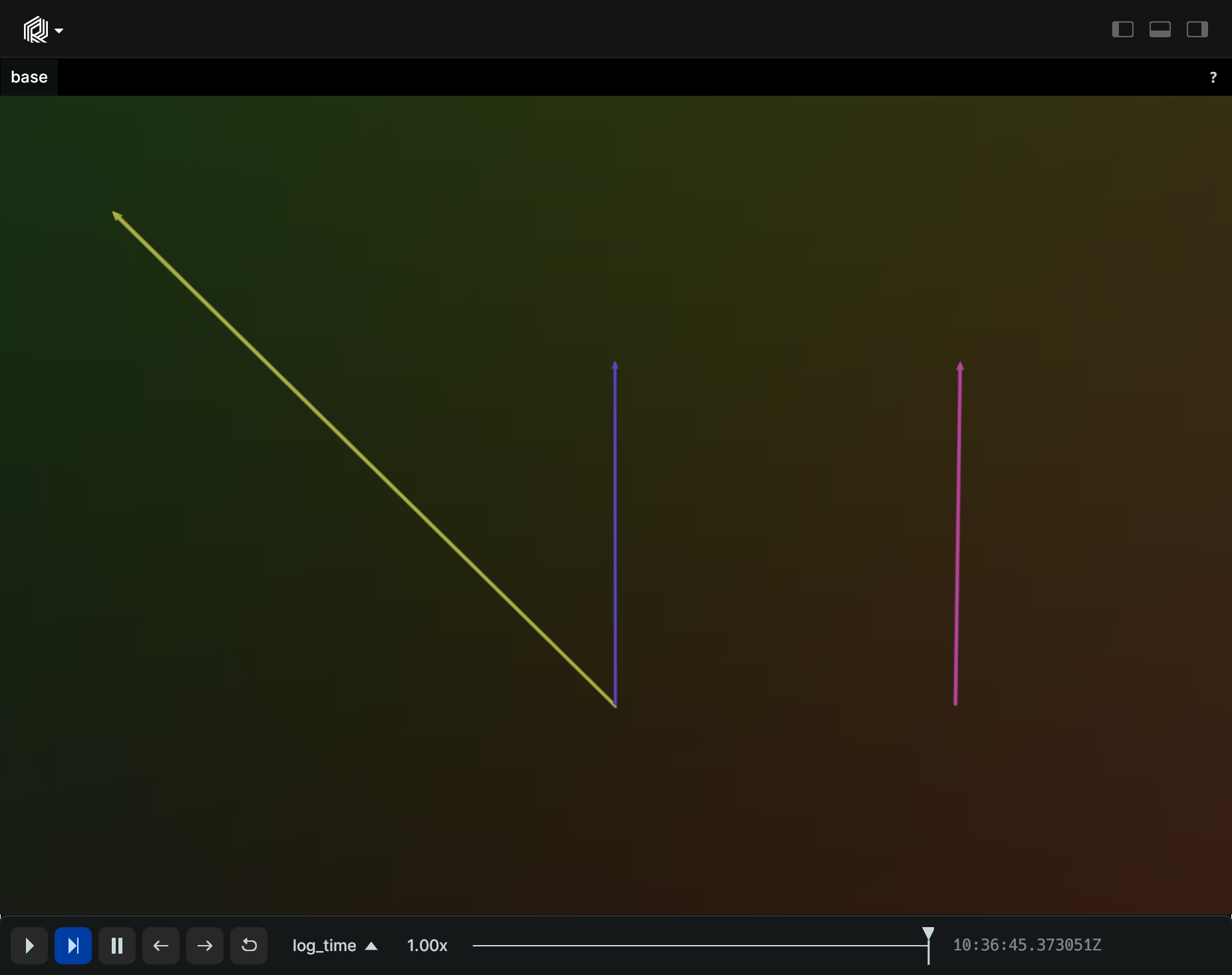# Transform3D

A 3D transform.

## Components

Required: `Transform3D`

## Example

### Variety of 3D transforms

``````"""Log different transforms between three arrows."""
from math import pi

import rerun as rr
from rerun.datatypes import Angle, RotationAxisAngle

rr.init("rerun_example_transform3d", spawn=True)

arrow = rr.Arrows3D(origins=[0, 0, 0], vectors=[0, 1, 0])

rr.log("base", arrow)

rr.log("base/translated", rr.Transform3D(translation=[1, 0, 0]))
rr.log("base/translated", arrow)

rr.log(
"base/rotated_scaled",
rr.Transform3D(
rotation=RotationAxisAngle(axis=[0, 0, 1], angle=Angle(rad=pi / 4)),
scale=2,
),
)
rr.log("base/rotated_scaled", arrow)
``````